U.S. Department of Transportation
1200 New Jersey Avenue, SE
Washington, DC 20590
202-366-4000

Federal Highway Administration Research and Technology
Coordinating, Developing, and Delivering Highway Transportation InnovationsThis report is an archived publication and may contain dated technical, contact, and link information
 Federal Highway Administration > Publications > Research Publications > 05081 > Design of Continuously Reinforced Concrete Pavements Using Glass Fiber Reinforced Polymer Rebars
 Publication Number: FHWA-HRT-05-081 Date: October 2005

# 508 Caption

Figure 1. Photo. Typical example of reinforcement layout for CRCP (constructed on S.R. 288 in Virginia). A steel-reinforced CRCP section constructed on S.R. 288 in Virginia utilizes a typical CRCP reinforcement design. This 25.4 centimeter (10 inch) thick CRCP consists of number 6 longitudinal reinforcement spaced at 15.24 centimeters (6 inches) and number 5 transverse reinforcement spaced at 121.9 centimeters (48 inches). A 7.62 centimeter (3 inch) thick asphalt stabilized open grade drainage layer (OGDL) over a 15.24 centimeter (6 inch) thick cement treated aggregate layer supports the CRCP.

Figure 2. Equation. A subscript s. A subscript s equals the product of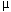times L times w, that product divided by the product of 2 times f subscript s.

Figure 3. Equation. A subscript f,sh. A subscript f,sh equals the product oftimes L times w, that product divided by the product of 2 times 0.0012 times E subscript f.

Figure 4. Equation. R. R equals the square root of the product of H times B, that product divided by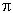.

Figure 5. Drawing. Schematic details of representative reinforced concrete prism. To estimate the development of shrinkage and thermal stresses in a free supported reinforced concrete slab, a representative concrete prismatic model containing a longitudinal reinforcing rebar at its center with width B, height H, length L, and rebar diameter 2rr, is considered.

Figure 6. Drawing. Schematic details of equivalent cylinder used in analyses. The model in figure 5 is modified into an equivalent cylinder with the corresponding equivalent diameter 2R accompanied by the same length and rebar diameter as those for the prismatic model.

Figure 7. Equation. dP/dx. Derivative of P with respect to x equals the product of C subscript o times the sum of u minus v.

Figure 8. Equation. τ(r). τ as a function of r equals the product of the derivative of P with respect to x times the inverse of the product of 2 timestimes r.

Figure 9. Equation. (u-v) in the form of integrating γ(r). The sum of u minus v equals the integration of γ, as a function of r, with respect to r when r varies from r subscript r to R, the integration of which equals the integration of the quotient of τ, as a function of r, divided by G subscript c with respect to r when r varies from r subscript r to R.

Figure 10. Equation. (u-v) in the solved form. The sum of u minus v equals the product of the derivative of P with respect to x times the quotient of the natural logarithm of the quotient of R divided r subscript r divided by the product of 2 timestimes G subscript c.

Figure 11. Equation. Co. C subscript o equals the quotient: the product of 2 timestimes G subscript c divided by the natural logarithm of the quotient of R divided by r subscript r.

Figure 12. Equation. d2P/dx2. The second derivative of P with respect to x equals the product: C subscript o times the sum of subscript r minus ε subscript c.

Figure 13. Equation. ε subscript c,s as a function of t. ε subscript c,s as a function of t equals the product of the quotient of negative t divided by the sum of 35 plus t times (ε subscript c,s) subscript ult; and subscript c,t equals the product: ΔT times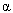subscript c.

Figure 14. Equation. ε subscript c,t. ε subscript c,t equals the product of T times subscript c.

Figure 15. Equation. ε subscript r,s. ε subscript r,s equals the quotient of P divided by the product of A subscript r times E subscript r.

Figure 16. Equation. ε subscript r,t subscript r,t equals the sum of the product of ΔT timessubscript r plus the quotient of P divided by the product of A subscript r times E subscript r.

Figure 17. Equation. (subscript c,s) subscript avg. (subscript c,s) subscript avg equals the product of ρ times E subscript r times the quotient of t divided by the sum of 35 plus t times (ε subscript c,s) subscript ult times the sum of 1 minus the quotient of the hyperbolic-cosine of the product of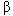times the sum of the quotient of L divided by 2 minus x divided by the hyperbolic cosine of the product oftimes the quotient of L divided by 2.

Figure 18. Equation. (subscript c,t) subscript avg. (subscript c,t) subscript avg equals the product of negative ρ times E subscript r times ΔT times the sum ofsubscript c minus subscript r times the sum of 1 minus the quotient of the hyperbolic-cosine of the product oftimes the sum of the quotient of L divided by 2 minus x divided by the hyperbolic cosine of the product oftimes the quotient of L divided by 2.

Figure 19. Equation..equals the square root of the quotient: the quotient of the product of 2 times G subscript c divided by E subscript r divided by the product of r, subscript r, raised to the second power times the natural logarithm of the quotient of R divided by r subscript r.

Figure 20. Equation. E subscript c, as a function of t when t equals or less than 28. E subscript c, as a function of t when t equals or less than 28, equals the product of E subscript c,28 times the sum of 0.52 plus the product of 0.15 times the natural logarithm of t.

Figure 21. Equation. E subscript c, when t is more than 28. E subscript c, when t is more than 28, equals the product of 1.019 times E subscript c,28.

Figure 22. Graph. Maximum average tensile stress in concrete versus time (ρ equals 0.00519 and L equals 1.524 meters (60 inches)). The maximum average tensile stresses in concrete due to the concrete shrinkage are calculated over a period of time. The maximum concrete stress level created by GFRP rebar is about one-fifth of that by steel rebar. Also, this figure shows a good agreement between the analytical and FE predictions of the maximum average tensile stress in concrete.

Figure 23. Graph. Maximum average tensile stress in concrete versus time (L equals 1.524 m (60 inches) and different GFRP reinforcing ratios, ρ). The cases for different sizes of GFRP rebars, numbers 3 through 6 (ρ equals 0.00184 through 0.00739), are presented, showing that the maximum concrete stress increases with an increase in the reinforcement ratio.

Figure 24. Graph. Maximum average tensile stress in concrete versus time (ρ equals 0.00519 GFRP and different slab lengths, L). The maximum concrete stresses for different slab lengths, L, are examined. The stresses increase with increases in length, L, increasingly converging to ρErεc,s(t) when L approaches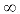. The maximum concrete stress already converges to ρErεc,s(t) as L reaches about 0.305 meter (12 inches).

Figure 25. Graph. Maximum average axial stress in concrete versus temperature change (ρ equals 0.00519 and L equals 1.524 meters (60 inches)). The plot of the maximum average axial stress in concrete versus temperature increase (positive ΔT) is shown for the case of granite aggregate concrete having a CTE of 10.26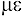per degree Celsius (5.7 per degree Fahrenheit). The CTE of concrete causes tensile concrete stress when using steel rebar and compressive concrete stress when using GFRP rebar as temperature increases.

Figure 26. Graph. Maximum average axial stress in concrete versus. temperature change (ρ equals 0.00519 and L equals 1.524 meters (60 inches)). The plot of the maximum average axial stress in concrete versus temperature increase (positive ΔT) is shown for the case of siliceous river gravel aggregate concrete having a CTE of 14.40 per degree Celsius (8.0per degree Fahrenheit). The CTE of concrete causes compressive stresses in concrete in both cases of using steel rebar and using GFRP rebar, as temperature increases. Also, this figure shows a good agreement between the analytical and FE predictions of the maximum average axial stress in concrete.

Figure 27. Graph. Axial stress in concrete versus. longitudinal location (comparison between analytical and FEM results). The axial concrete stress distribution along the longitudinal (x-) direction for a GFRP-reinforced slab with a temperature variation of negative 27.78 degree Celsius (negative 50 degrees Fahrenheit) is calculated using both analytical and FE methods. The figure shows that the axial stresses from the analytical model, which are averaged in the radial (r-) direction, agree well with the axial stresses from all the layers of the concrete FE element as the x-location approaches the middle of the section. From the FE results, it can also be seen that the axial stresses are varied through the depth around the ends of the slab.

Figure 28. Equation. (ε subscript c) subscript gage. (ε subscript c) subscript gage equals the sum of ε subscript c plus (ε subscript c) subscript avg.

Figure 29. Equation. (ε subscript c,s) subscript gage. (ε subscript c,s) subscript gage equals the product of the quotient of negative t divided by the sum of 35 plus t times (ε subscript c,s) subscript ult times the sum of 1 minus the product of times the quotient of E subscript r divided by E subscript c, as a function of t, times the sum of 1 minus the quotient of the hyperbolic cosine of the product of β times the sum of the quotient of L divided by 2 minus x divided by the hyperbolic-cosine of the product of β times the quotient of L divided by 2.

Figure 30. Equation. (ε subscript c,t) subscript gage. (ε subscript c,t) subscript gage equals the product of ΔT times the sum of subscript c minus the product of ρ times the quotient of E subscript r divided by E subscript c times the sum of subscript c minus subscript r times the sum of 1 minus the quotient of the hyperbolic-cosine of the product of β times the sum of the quotient of L divided by 2 minus x divided by the hyperbolic-cosine of the product of β times the quotient of L divided by 2.

Figure 31. Photo. Specimen for CTE measurement of number 4 steel rebar. A strain gage (model EA-06-125BB-120, manufactured by Measurement Group, Inc.) is attached at the middle of the steel rebar in the longitudinal direction in order to measure the CTEs of the steel and GFRP rebars.

Figure 32. Photo. Specimen for CTE measurement of number 4 GFRP rebar. A strain gage (model EA-06-125BB-120 manufactured by Measurement Group, Inc.) is attached at the middle of the GFRP rebar in the longitudinal direction in order to measure the CTEs of the steel and GFRP rebars.

Figure 33. Photo. Specimen for CTE measurement of titanium silicate strip. A strain gage (model EA-06-125BB-120 manufactured by Measurement Group, Inc.) is attached at the middle of the titanium silicate strip in order to measure the CTEs of the steel and GFRP rebars.

Figure 34. Equation. The sum ofsubscript T/M minussubscript R/M. The sum ofsubscript T/M minus subscript R/M equals the quotient of the sum of (ε subscript T/O) subscript T/M minus (ε subscript T/O) subscript R/M divided by ΔT.

Figure 35. Equation.subscript T/M times ΔT.subscript T/M times ΔT equals the sum of (ε subscript T/O) subscript T/M minus (ε subscript T/O) subscript R/M.

Figure 36. Graph. Coefficient of thermal expansion versus temperature for reinforcing rebars from third measurement. The figure shows that the CTE of each rebar is fairly consistent at different applied temperature levels. The average CTEs obtained are about 11.88per degree Celsius (6.6per degree Fahrenheit) for the steel rebar and 9.18per degree Celsius (5.1per degree Fahrenheit) for the GFRP rebar.

Figure 37. Drawing. Schematic details of concrete slabs used in experiment. The schematic details of the concrete slab specimens are shown. The three laboratory slab specimens, a plain concrete slab, a steel (number 4 rebar with a radius of 6.35 mm (0.25 inch) and ρ equals 0.01695) reinforced concrete slab, and a GFRP (number 4 rebar) reinforced concrete slab, are cast. A thermocouple is set in the center of each slab before the concrete cast, and a strain gage (model N2A-06-40CBY-120 manufactured by Measurement Group, Inc.) is attached on the center of the upper surface of each slab after the curing process. Each reinforced slab includes three reinforcing rebars. The specimens all have dimensions of 0.458 meter by 0.915 meter by 50.8 millimeters (1.5 feet by 3 feet by 2 inches).

Figure 38. Photo. Concrete slabs used in experiment: slab molds before concrete cast. The figure shows the photos of the molds of concrete slabs. The slabs are subjected to cyclic temperature changes in an environmental chamber with temperature and humidity control.

Figure 39. Photo. Concrete slabs used in experiment: slabs attached with strain gages. The figure shows the photos of the concrete slabs prior to the experiment. The slabs are subjected to cyclic temperature changes in an environmental chamber with temperature and humidity control.

Figure 40. Graph. Axial concrete strain at midspan versus. temperature change from analytical calculation. In the figure, it is predicted that the axial concrete strains calculated for the steel-reinforced slab have higher contraction magnitudes, shown as negative values, than those for the plain and GFRP-reinforced slabs under temperature drop.

Figure 41. Graph. Axial concrete strain at midspan versus temperature change for plain concrete slab, and reference temperature of T subscript o equals about 42.39 degree Celsius (108.3 degrees Fahrenheit). The axial concrete strains measured at the midspan of each slab over the temperature changes ranging from T equals positive 49 degree Celsius to negative 29 degree Celsius (positive 120.2 degrees Fahrenheit to negative 20.2 degrees Fahrenheit) are compared with the analytical calculations. The measured strains under freezing temperatures seem to have contraction magnitudes that are somewhat smaller than the calculated values.

Figure 42. Graph. Axial concrete strain at midspan versus temperature change for steel-reinforced concrete slab, and reference temperature of T subscript o equals about 42.39 degree Celsius (108.3 degrees Fahrenheit). The axial concrete strains measured at the midspan of each slab over the temperature changes ranging from T equals positive 49 degree Celsius to negative 29 degree Celsius (positive 120.2 degrees Fahrenheit to negative 20.2 degrees Fahrenheit) are compared with the analytical calculations. The measured strains under freezing temperatures seem to have contraction magnitudes that are somewhat smaller than the calculated values.

Figure 43. Graph. Axial concrete strain at midspan versus temperature change for GFRP-reinforced concrete slab, and reference temperature of T subscript o equals about 42.39 degree Celsius (108.3 degrees Fahrenheit). The axial concrete strains measured at the midspan of each slab over the temperature changes ranging from T equals positive 49 degree Celsius to negative 29 degree Celsius (positive 120.2 degrees Fahrenheit to negative 20.2 degrees Fahrenheit) are compared with the analytical calculations. The measured strains under freezing temperatures seem to have contraction magnitudes that are somewhat smaller than the calculated values.

Figure 44. Drawing. Schematic details of a 2-D CRCP finite element model. The concrete slab segment is modeled as four-node, 2-D elements under plane stress conditions, and the reinforcement is modeled as beam elements with circular cross sections. The bond-slip behaviors between the concrete and reinforcement and between the concrete slab and subbase were modeled by using horizontal spring elements. The underlying layers are modeled as an elastic foundation. The length of the slab-segment, L, is equal to the crack spacing. The FE model of CRCP slab-segment has been assumed to be subjected to a concrete shrinkage and the temperature change. The temperature varies linearly from the top to the bottom.

Figure 45. Graph. Axial tensile stress on concrete surface versus longitudinal location of a 1.524 meter (5 foot) CRCP segment with ρ equal to 0.00739 andsubscript c equal to 10.26per degree Celsius (5.7per degree Fahrenheit). Because of the low Young's modulus in the GFRP rebar, the tensile stresses for the GFRP-reinforced concrete are always smaller than those for the steel ones. The maximum tensile stresses in concrete for both steel and GFRP reinforced slab segments are much higher than the concrete tensile strength, and all the slab segments will presumably be cracked at its midspan.

Figure 46. Graph. Axial tensile stress on concrete surface versus. longitudinal location of a 1.524 meter (5 foot) CRCP segment with ρ equal to 0.00739 andsubscript c equal to 14.40per degree Celsius (8.0per degree Fahrenheit). The tensile stresses for the GFRP-reinforced concrete are smaller than those for the steel ones. The maximum tensile stresses in concrete for both steel and GFRP reinforced slab-segments are much higher than the concrete tensile strength, and all the slab segments will presumably be cracked at its mid-span. In the comparison between Figures 45 and 46, it can be observed that the tensile stress level in concrete for the siliceous river gravel concrete with a CTE of 14.40per degree Celsius (8.0per degree Fahrenheit) is higher than that for the granite concrete with a CTE of 10.26per degree Celsius (5.7per degree Fahrenheit).

Figure 47. Graph. Axial stress in reinforcement versus longitudinal location of a 1.524 meter (5 foot) CRCP segment with ρ equal to 0.00739 andsubscript c equal to 10.26 per degree Celsius (5.7per degree Fahrenheit). While the reinforcements around the middle are under low compression, those around the crack (the ends of the slab segment) are under high tension; the maximum tensile stress in steel reinforcement is about 661.92 MPa (96 kip per square inch), and that in GFRP reinforcement is about 234.43 MPa (34 kip per square inch). Because of the stiffness compatibility between GFRP and concrete, the tensile stresses in the GFRP reinforcements are lower than those in the steel reinforcements.

Figure 48. Graph. Axial stress in reinforcement versus longitudinal location of a 1.524 meter (5 foot) CRCP segment with ρ equal to 0.00739 andsubscript c equal to 14.40 per degree Celsius (8.0per degree Fahrenheit). While the reinforcements around the middle are under low compression, those around the crack (the ends of the slab segment) are under high tension; the maximum tensile stress in steel reinforcement is about 765.35 MPa (111 kip per square inch), and that in GFRP reinforcement is about 275.8 MPa (40 kip per square inch). The tensile reinforcement stresses for the higher concrete CTE (Figure 48) are higher than those for the lower concrete CTE (Figure 47).

Figure 49. Graph. Axial stress at concrete bottom surface versus longitudinal location for two different subbase bond stiffnesses (ρ equals 0.00739 and L equals 1.524 meters (60 inches)). The figure shows the FEM results of concrete stresses at the bottom surface of the GFRP-reinforced concrete pavement along the longitudinal (x-) direction, from varying the bond-slip stiffness between the concrete and subbase from 0.236 MN per meter to 24.271 MN per meter (1,350 poundsforce per inch. to 138,600 poundsforce per inch). The tensile stresses in the concrete for the higher subbase friction are larger than those for the lower one.

Figure 50. Graph. Axial stress at concrete top surface versus longitudinal location for two different subbase bond stiffnesses (ρ equals 0.00739 and L equals 1.524 meters (60 inches)). The figure shows the FEM results of concrete stresses at the top surface of the GFRP-reinforced concrete pavement along the longitudinal (x-) direction, from varying the bond-slip stiffness between the concrete and subbase from 0.236 MN per meter to 24.271 MN per meter (1,350 poundsforce per inch. to 138,600 poundsforce per inch). At the top of the slab segment, the smaller stresses were found for the higher friction case, which is the result opposite of that in Figure 49.

Figure 51. Image. Axial stress in concrete versus longitudinal location of a 1.524 meter (5 foot) CRCP segment with ρ equal to 0.00739, subscript c equal to 10.26per degree Celsius (5.7per degree Fahrenheit), and a subbase bond-slip stiffness of 0.236 MN per meter (1,350 poundsforce per inch). This figure, representing the lower friction case, shows that the stress variation is almost symmetrical with respect to the longitudinal center line, on which the reinforcement is located.

Figure 52. Image. Axial stress in concrete versus longitudinal location of a 1.524 meter (5 foot) CRCP segment with equal to 0.00739, subscript c equal to 10.26per degree Celsius (5.7per degree Fahrenheit), and a subbase bond-slip stiffness of 24.271 MN per meter (138,600 poundsforce per inch). The stress in this figure, for the higher friction case, is observed to drastically change from the bottom to the top of the slab segment; the stress level at the bottom is much higher than that at the top. With such a high subbase friction, the CRCP is likely to have a chance to crack from its bottom area.

Figure 53. Graph. Axial stress in GFRP rebar versus longitudinal location for two different subbase bond stiffnesses (ρ equals 0.00739 and L equals 1.524 meters (60 inches)). The stress distributions in the GFRP reinforcement for the two different subbase friction cases (0.236 MN per meter to 24.271 MN per meter (1,350 poundsforce per inch and 138,600 poundsforce per inch)) are plotted in this figure. The compressive stresses (negative value in the figure) are developed around the middle of the slab segment in the longitudinal (x-) direction for both friction cases. The compressive stress level for the higher friction, about 6.48 MPa (0.94 kip per square inch), is slightly lower than that for the lower friction, about 7.79 MPa (1.13 kip per square inch). At the cracks, a maximum tensile stress of about 222.29 MPa (32.24 kip per square inch) is developed for the higher friction and is also lower than that of about 237.12 MPa (34.39 kip per square inch) for the lower friction.

Figure 54. Graph. Pavement depth versus crack width for two different subbase bond stiffnesses (ρ equals 0.00739 and L equals 1.524 meters (60 inches)). The variations of crack width through the depth of the slab segment for the two different subbase friction cases (0.236 MN per meter to 24.271 MN per meter (1,350 poundsforce per inch and 138,600 poundsforce per inch)) are observed in this figure. The crack width at the bottom for the higher friction (0.432 mm (0.017 inch)) is narrower than that for the lower friction (0.584 mm (0.023 inch)) due to more restraint from the subbase. The crack width for the higher friction is narrower up to about middepth of the slab, where the reinforcement is located, and becomes wider at the top (0.813 mm (0.032 inch)), presenting more drastic variations through the depth. In the figure, the protrusion on the graph is found at the middepth due to the restraint from the reinforcement, and the crack width at the top of the pavement is shown to be wider than that at the bottom, since the magnitude of the temperature drop ( T) is larger at the top than at the bottom and the restraint from subbase exists at the bottom.

Figure 55. Graph. Axial tensile stress on concrete surface versus longitudinal location for different bond stiffnesses (ρ equals 0.00739 and L equals 1.524 meters (60 inches)). The figure shows that the concrete stress level from a larger bond-slip stiffness is higher than that from a smaller stiffness since the tighter bond between the concrete and reinforcement provides more restraint to the concrete's volume change. The bond-break near the crack causes the tensile concrete stress to decrease from about 0.92 kip per square inch to 6.34 MPa to 4.14 MPa (0.60 kip per square inch) on the concrete surface.

Figure 56. Graph. Axial stress in GFRP rebar versus longitudinal location for different bond stiffnesses (ρ equals 0.00739 and L equals 1.524 meters (60 inches)). The figure shows that the stress level around the crack decreases as the bond-slip stiffness decreases. In addition, the bond-break near the crack increases the tensile reinforcement stress from about 34.39 kip per square inch to 237.12 MPa to 285.94 MPa (41.47 kip per square inch) at the cracks and the compressive reinforcement stress from about 1.13 kip per square inch to 7.79 MPa to 10.48 MPa (1.52 kip per square inch) at the midspan.

Figure 57. Graph. Pavement depth versus crack width for different bond stiffnesses (ρ equals 0.00739 and L equals 1.524 meters (60 inches)). The crack width for the higher bond-slip stiffness is narrower than that for the lower ones as a result of having more restraint against the concrete volume change. Also, the crack width for steel reinforcement is narrower than that for GFRP reinforcement at a bond-slip stiffness due to the higher Young's modulus of the steel reinforcement. The graph is observed to be more protruded with the higher bond-slip stiffness. This is due to the fact that the concrete volume change is subjected to more restraint as the bond-slip stiffness increases. In addition, the effect of the bond-break on the crack width variation in the perfect bond case of GFRP reinforcement is shown in the figure. Since the bond breaks near the crack, the crack widths throughout the pavement depth become wider than those without the bond-break assumption.

Figure 58. Image. Axial stress in concrete versus longitudinal location of a 1.524 meter (60 inches) CRCP segment with equal to 0.00739, subscript c equal to 10.26per degree Celsius (5.7 per degree Fahrenheit), and a subbase bond-slip stiffness of 0.236 MN per meter (1,350 poundsforce per inch; bond-break considered). The bond-break between the concrete and reinforcement near the crack relieves the stress concentration. In this FE simulation, the bond-slip springs between concrete and reinforcement are modeled to yield when the relative spring displacement between concrete and reinforcement exceeds 0.004 inch (1 millimeter). The calculated bond-break appears at the first spring element; hence, the bond-break length is less than 1.5 inches starting from the surface of the crack. The bond-break near the crack also lowers the overall stress level in concrete.

Figure 59. Graph. Axial tensile stress on concrete surface versus longitudinal location for different slab segment lengths (ρ equal to 0.00739; bond-slip stiffness equall to 433.24 MPa per meter (2.474 times 106 poundsforce per inch)). The CRCP segments at three different lengths of 1.067 meters (3.5 feet), 1.524 meters (5 feet), and 2.438 meters (8 feet) are modeled to represent the range of the crack spacing anticipated from the conventional CRCP design, which is 1.067 meters (3.5 feet) to 2.438 meters (8 feet). The concrete stress level of the longer slab segment is higher than that of the shorter slab segment. The maximum stress levels in the concrete for the lengths of 1.067 meters (3.5 feet), 1.524 meters (5 feet), and 2.438 meters (8 feet) are about 1.86 MPa (270 pounds per square inch), 3.08 MPa (447 pounds per square inch), and 6.16 MPa (893 pounds per square inch), respectively.

Figure 60. Graph. Axial stress in GFRP rebar versus longitudinal location for different slab segment lengths (ρ equal to 0.00739 and bond-slip stiffness equal to 433.24 MPa per meter (2.474 times 106 poundsforce per inch)). Around the crack, the stress levels in the GFRP reach around 113.83 MPa (16.51 kip per square inch), 192.70 MPa (27.95 kip per square inch), and 387.59 MPa (56.21 kip per square inch) for the lengths of 1.067 meters (3.5 feet), 1.524 meters (5 feet), and 2.438 meters (8 feet), respectively.

Figure 61. Graph. Pavement depth versus crack width for different slab segment lengths (ρ equal to 0.00739 and bond-slip stiffness equal to 433.24 MPa per meter (2.474 times 106 poundsforce per inch)). The crack width for the longer slab segment is wider than that for the shorter one. The crack widths at the top of the slab are about 0.406, 0.686, and 1.321 mm (0.016, 0.027, and 0.052 inch) for the slab segment lengths of 1.067, 1.524, and 2.438 meter (3.5, 5, and 8 feet), respectively.

Figure 62. Graph. Mean crack spacing versus type of coarse aggregate in concrete mix (ρ equals to 0.0074, asphalt-stabilized subbase). The figure shows the predicted mean crack spacings over various concrete coarse aggregate types as follows: 1.814 meter (5.95 feet) for limestone, 2.457 meter (8.06 feet) for basalt, 2.115 meter (6.94 feet) for granite, 1.058 meter (3.47 feet) for granite for steel-reinforced CRCP, 1.905 meter (6.25 feet) for dolomite, 1.359 meter (4.46 feet) for sandstone, 1.905 meter (6.25 feet) for quartz, and 1.003 meter (3.29 feet) for siliceous river gravel.

Figure 63. Graph. Crack width versus type of coarse aggregate in concrete mix (ρ equals to 0074, asphalt-stabilized subbase). The figure shows the predicted crack widths over various concrete coarse aggregate types as follows: 0.54 mm (0.0211 inch) for limestone, 0.93 mm (0.0365 inch) for basalt, 0.99 mm (0.0388 inch) for granite, 0.49 mm (0.0193 inch) for granite for steel-reinforced CRCP, 0.92 mm (0.0361 inch) for dolomite, 0.72 mm (0.0283 inch) for sandstone, 1.02 mm (0.0402 inch) for quartz, and 0.67 mm (0.0263 inch) for siliceous river gravel. In general, the crack widths appear to be wider as their corresponding crack spacings are larger. Also, a larger volume change in concrete appears to contribute in developing wider crack width.

Figure 64. Graph. Tensile stress in reinforcement at crack versus type of coarse aggregate in concrete mix (ρ equals to 0.0074, asphalt-stabilized subbase). The figure shows the predicted tensile stress level in reinforcement at crack for various types of concrete coarse aggregate as follows: 137.15 MPa (19.891 kip per square inch) for limestone, 173.58 MPa (25.175 kip per square inch) for basalt, 175.59 MPa (25.466 kip per square inch for granite), 288.56 MPa (41.850 kip per square inch) for granite for steel-reinforced CRCP, 167.89 MPa (24.350 kip per square inch) for dolomite, 147.84 MPa (21.441 kip per square inch) for sandstone, 176.26 MPa (25.564 kip per square inch) for quartz, and 135.73 MPa (19.686 kip per square inch) for siliceous river gravel. The stress levels in GFRP-reinforcement appear to correlate with their corresponding crack widths.

Figure 65. Graph. Mean crack spacing and crack width versus bond-slip stiffness/unit area (ρ equals 0.0074 for granite aggregate concrete). The figure shows the predicted mean crack spacing and crack width over the bond-slip stiffness/unit area as follows: 2.518 meter (8.26 feet) and 1.21 mm (0.0478 inch) for 5.958 MPa per meter (21.95 poundsforce per square inch per inch), 2.115 meter (6.94 feet) and 0.99 mm (0.0388 inch) for 15.169 MPa per meter (55.88 poundsforce per square inch per inch), 2.115 meter (6.94 feet) and 0.99 mm (0.0388 inch) for 39.483 MPa per meter (145.45 poundsforce per square inch per inch), 2.060 meter (6.76 feet) and 0.96 mm (0.0378 inch) for 41.954 MPa per meter (154.55 poundsforce per square inch per inch), 1.814 meter (5.95 feet) and 0.85 mm (0.0334 inch) for 0.136 GPa per meter (500 poundsforce per square inch per inch), 1.731 meter (5.68 feet) and 0.81 mm (0.0318 inch) for 0.271 GPa per meter (1,000 poundsforce per square inch per inch), 1.414 meter (4.64 feet) and 0.66 mm (0.0260 inch) for 0.543 GPa per meter (2,000 poundsforce per square inch per inch), 1.088 meter (3.57 feet) and 0.51mm (0.0201 inch) for 1.357 GPa per meter (5,000 poundsforce per square inch per inch), 0.887 meter (2.91 feet) and 0.42 mm (0.0164 inch) for 2.715 GPa per meter (10,000 poundsforce per square inch per inch), and 0.747 meter (2.45 feet) and 0.35 mm (0.0139 inch) for 4.180 GPa per meter (15,400 poundsforce per square inch per inch). From the figure, it can be seen that the higher bond-slip results in smaller crack spacing, which in turn causes narrower crack widths.

Figure 66. Graph. Tensile stress in GFRP reinforcement at crack versus bond-slip stiffness/unit area (ρ equals 0.0074 for granite aggregate concrete). The figure shows the predicted tensile stress level in GFRP reinforcement at crack over the bond-slip stiffness/unit area as follows: 196.51 MPa (28.501 kip per square inch) for 5.958 MPa per meter (21.95 poundsforce per square inch per inch), 175.59 MPa (25.466 kip per square inch) for 15.169 MPa per meter (55.88 poundsforce per square inch per inch), 175.60 MPa (25.468 kip per square inch) for 39.483 MPa per meter (145.45 poundsforce per square inch per inch), 170.78 MPa (24.769 kip per square inch) for 41.954 MPa per meter (154.55 poundsforce per square inch per inch), 160.34 MPa (23.255 kip per square inch) for 0.136 GPa per meter (500 poundsforce per square inch per inch), 157.85 MPa (22.893 kip per square inch) for 0.271 GPa per meter (1,000 poundsforce per square inch per inch), 144.02 MPa (20.887 kip per square inch) for 0.543 GPa per meter (2,000 poundsforce per square inch per inch), 125.88 MPa (18.256 kip per square inch) for 1.357 GPa per meter (5,000 poundsforce per square inch per inch), 112.04 MPa (16.250 kip per square inch) for 2.715 GPa per meter (10,000 poundsforce per square inch per inch), and 102.54 MPa (14.871 kip per square inch) for 4.180 GPa per meter (15,400 poundsforce per square inch per inch). From the figure, it can be seen that the higher bond-slip causes lower tensile stress in the reinforcement at the crack, since the smaller crack spacing is expected with the higher bond-slip.